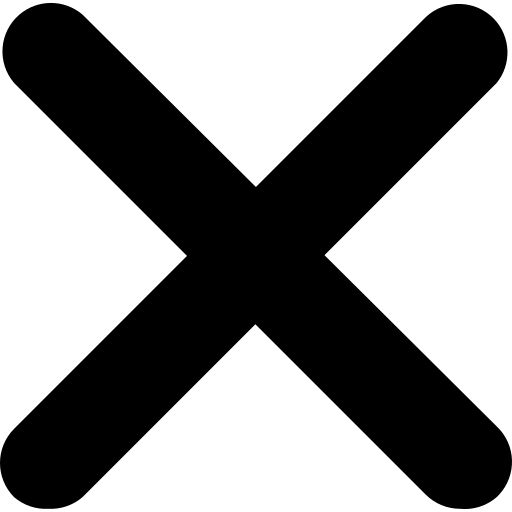#### Are You Looking for Developers to Hire?Have a Look at Our Bench Strength# 50 Common Java Coding Interview Questions

By Arun Kumar1. What is Java?
2. What are the main features of Java?
3. What is the difference between JDK, JRE, and JVM?
4. What is OOP?
5. What is the difference between abstract class and interface?
6. What is inheritance?
7. What is polymorphism?
8. What is encapsulation?
9. What is the difference between private, protected, and public access modifiers?
10. What is a static method?
11. What is a final class?
12. What is a final variable?
13. What is the difference between == and equals() method?
14. What is the difference between checked and unchecked exceptions?
15. What is a Java package?
16. What is a Java classpath?
17. What is a constructor?
18. What is a garbage collector?
20. What is synchronization?
21. What is a volatile variable?
22. What is a transient variable?
23. What is a static block?
24. What is the difference between StringBuffer and StringBuilder?
25. What is a lambda expression?
26. What is the difference between anonymous class and lambda expression?
27. What is a functional interface?
28. What is a stream in Java 8?
29. What is the difference between stream and collection?
30. What is the difference between map() and flatMap() methods in Java 8?
31. What is the difference between parallelStream() and stream() in Java 8?
32. What is a default method in Java 8?
33. What is a method reference?
34. What is the diamond problem in Java?
35. What is a singleton class?
36. What is the difference between an ArrayList and a LinkedList?
37. What is the difference between a HashSet and a TreeSet?
38. What is the difference between a HashMap and a TreeMap?
39. What is the difference between an inner class and a nested class?
40. What is a reflection in Java?
41. What is a proxy in Java?
42. What is a JAXB?
43. What is JDBC?
44. What is Hibernate?
45. What is Spring Framework?
46. What is a design pattern in Java?
47. What is the difference between fail-fast and fail-safe iterators?
48. What is the difference between C++ and Java?
49. What is a JIT compiler?
50. What is the difference between a stack and a queue?